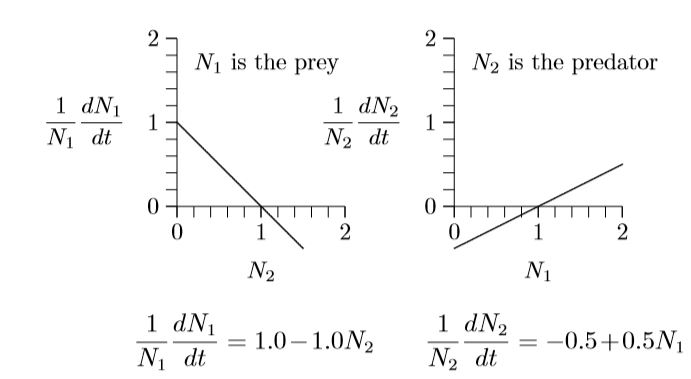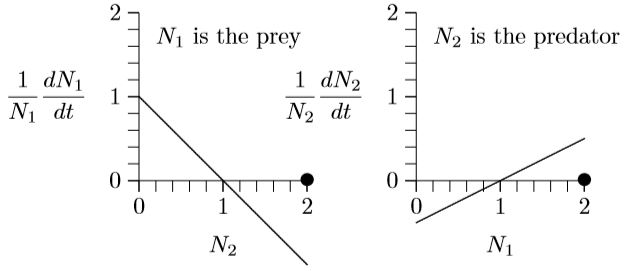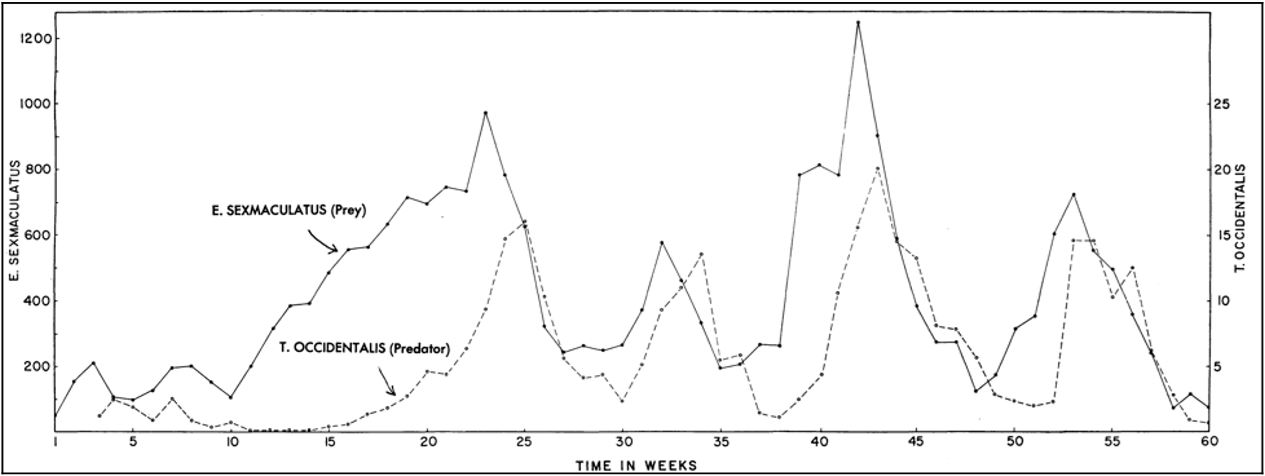# 12.3: Predator-prey model

$$\newcommand{\vecs}{\overset { \rightharpoonup} {\mathbf{#1}} }$$ $$\newcommand{\vecd}{\overset{-\!-\!\rightharpoonup}{\vphantom{a}\smash {#1}}}$$$$\newcommand{\id}{\mathrm{id}}$$ $$\newcommand{\Span}{\mathrm{span}}$$ $$\newcommand{\kernel}{\mathrm{null}\,}$$ $$\newcommand{\range}{\mathrm{range}\,}$$ $$\newcommand{\RealPart}{\mathrm{Re}}$$ $$\newcommand{\ImaginaryPart}{\mathrm{Im}}$$ $$\newcommand{\Argument}{\mathrm{Arg}}$$ $$\newcommand{\norm}{\| #1 \|}$$ $$\newcommand{\inner}{\langle #1, #2 \rangle}$$ $$\newcommand{\Span}{\mathrm{span}}$$ $$\newcommand{\id}{\mathrm{id}}$$ $$\newcommand{\Span}{\mathrm{span}}$$ $$\newcommand{\kernel}{\mathrm{null}\,}$$ $$\newcommand{\range}{\mathrm{range}\,}$$ $$\newcommand{\RealPart}{\mathrm{Re}}$$ $$\newcommand{\ImaginaryPart}{\mathrm{Im}}$$ $$\newcommand{\Argument}{\mathrm{Arg}}$$ $$\newcommand{\norm}{\| #1 \|}$$ $$\newcommand{\inner}{\langle #1, #2 \rangle}$$ $$\newcommand{\Span}{\mathrm{span}}$$

For the next several chapters we will consider two species, starting with one predator and one prey. Figure $$\PageIndex{1}$$ depicts this situation, with one line sloping down and the other up.Figure $$\PageIndex{1}$$. Predator–prey interactions with corresponding equations.

The graph on the left describes the prey, because its numbers N1 are reduced when the numbers of predator, N2, increase. Likewise, the graph on the right describes the predator, because its numbers, N2, increase with the density of its prey, N1. The equations of growth are revealed by the slopes and intercepts of the two lines.

Since these are both straight lines, $$y\,=\,mx\,+\,b$$, the equations can be written down simply from the geometry. The intercept on the left is +1 and the slope is −1. The intercept on the right is −1/2 and its slope is +1/2. The equivalent equations of the two lines appear below the graphs.

These specific equations can be generalized using symbols in place of actual numbers, writing r1, s1,2, r2, and s2,1 for the intercept +1.0 and slope −1.0 on the left and the intercept −0.5 and slope +0.5 on the right, as follows.

$$\frac{1}{N_1}\frac{dN_1}{dt}\,=\,r_1\,+\,s_{1,2}N_2\,\,\,\,\,\,\,with\,r_1\,=\,+\,1.0,\,\,\,s_{1,2}\,=\,-\,1.0$$

$$\frac{1}{N_2}\frac{dN_2}{dt}\,=\,r_2\,+\,s_{2,1}N_1\,\,\,\,\,\,\,with\,r_2\,=\,-\,0.5,\,\,\,s_{2,1}\,=\,+\,0.5$$

Merely by writing down the form of these geometric graphs, the classic Lotka–Volterra predator–prey equations have appeared:

$$\frac{1}{N_1}\frac{dN_1}{dt}\,=\,r_1\,+\,s_{1,2}N_2\,\,\,\,\,\,\,with\,r_1\,>\,0,\,\,\,s_{1,2}\,<\,0$$

$$\frac{1}{N_2}\frac{dN_2}{dt}\,=\,r_2\,+\,s_{2,1}N_1\,\,\,\,\,\,\,with\,r_2\,<\,0,\,\,\,s_{2,1}\,>\,0$$

Here is how the equations look in many textbooks, with V for prey density and P for predator density:

$$\frac{dV}{dt}\,=\,rV\,-\alpha\,VP$$

$$\frac{dP}{dt}\,=\beta\,VP\,-\,qP$$

Volterra arrived at the equation rather differently than we did, with a growth rate r for the prey, reduced by a rate $$\alpha$$ for each encounter between predator and prey, $$V\,\cdot\,P$$, and with a natural death rate q for predators and compensatory growth rate $$\beta$$ for each encounter,$$V\,\cdot\,P$$, between predator and prey.

To see the equivalence, divide the first equation through by V and the second by P, then set $$V\,=\,N_1,\,\,P\,=\,N_2,\,\,r\,=\,r_1,\,\,q\,=\,-r_2,\,\,\alpha\,=\,-s_{1,2},\,\,\beta\,=\,s_{2,1}$$. The Lotka–Volterra formulation will be revealed to be just the r + sN equations in disguise.

Figure $$\PageIndex{1}$$ exposes the basic predator–prey equations from geometry, which reveal the unity of the equations of ecology, as you saw in Chapter 5. That analysis revealed a form of one-dimensional equation not considered in ecological textbooks—the orthologistic equation—and which is needed for understanding human and other rapidly growing populations.

Now analyze these equations a bit. Suppose predator and prey densities are both 1, say 1 individual per hectare (N1 = N2 = 1). Substitute 1 for both N1 and N2. What are the growth rates?

$$\frac{1}{1}\frac{dN_1}{dt}\,=\,1.0\,-\,1.0\,\times\,1\,=\,0$$

$$\frac{1}{1}\frac{dN_2}{dt}\,=\,-0.5\,+\,0.5\,\times\,1\,=\,0$$

The population growth is zero for both species, so the populations do not change. This is an equilibrium.

This can be seen in the graphs below. The fact that both growth rates, $$\frac{1}{N_1}\frac{dN_1}{dt}$$ and $$\frac{1}{N_1}\frac{dN_2}{dt}$$, cross the horizontal axis at N1 = N2 = 1 (position of the dots) means that growth stops for both. This is called an equilibrium, a steady state, or, sometimes, a fixed point.But what will happen if both populations are 2, say 2 individuals per hectare?The prey growth rate, $$\frac{1}{N_1}\frac{dN_1}{dt}$$, is negative at N2 = 2 (the line is below the horizontal axis) and the predator growth rate, $$\frac{1}{N_1}\frac{dN_2}{dt}, is positive at N1 = 2 (the line is above the horizontal axis). So the prey population will decrease and the predator population will increase. Exactly how the populations will develop over time can be worked out by putting these parameters into the program in Chapter 8. Here it what it shows.Figure 12.5 as calculated by Program 95. For comparison, here is what early experimenters such as Gause and Huffaker showed for populations of protozoa, mites, and other small systems in the middle of the twentieth century:Figure \(\PageIndex{3}$$. Dynamics in an experimental predator–prey system conducted by C. B. Huffaker in the 1950s with two species of mite.

The dynamics here are much the same as those shown in the calculated version of Figure $$\PageIndex{2}$$ and the experimental version of Figure $$\PageIndex{3}$$, but with stochasticity overlayed on the experimental system. Experimenters, however, had difficulty achieving continual cycling. In simple conditions, the predators would find the prey and eat every last one, and then the predators themselves would all die. Continual cycling could be achieved by providing the prey with places to escape, or making it difficult for the predators to move around the environment.

This page titled 12.3: Predator-prey model is shared under a CC BY-NC 4.0 license and was authored, remixed, and/or curated by Clarence Lehman, Shelby Loberg, & Adam Clark (University of Minnesota Libraries Publishing) via source content that was edited to the style and standards of the LibreTexts platform; a detailed edit history is available upon request.